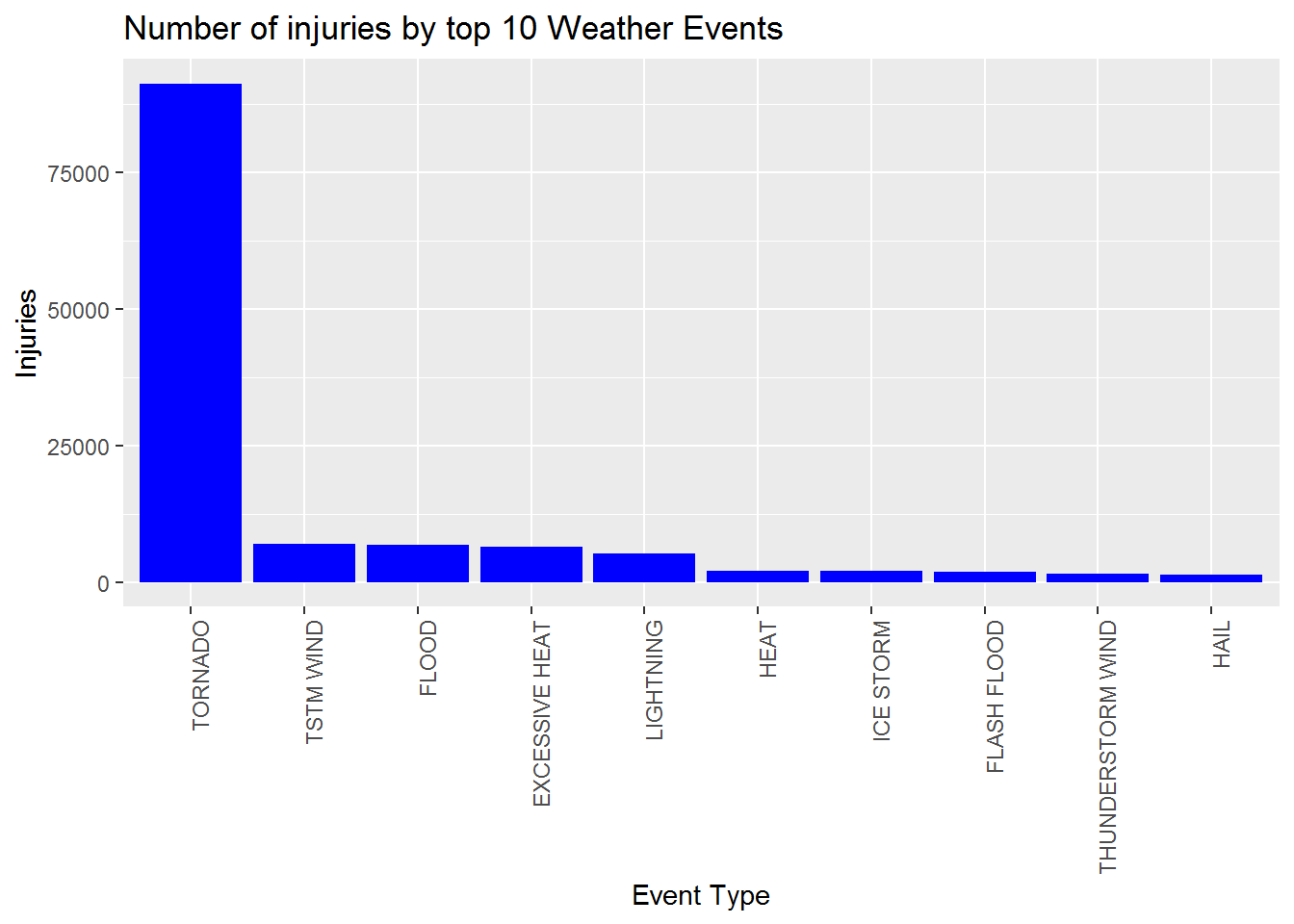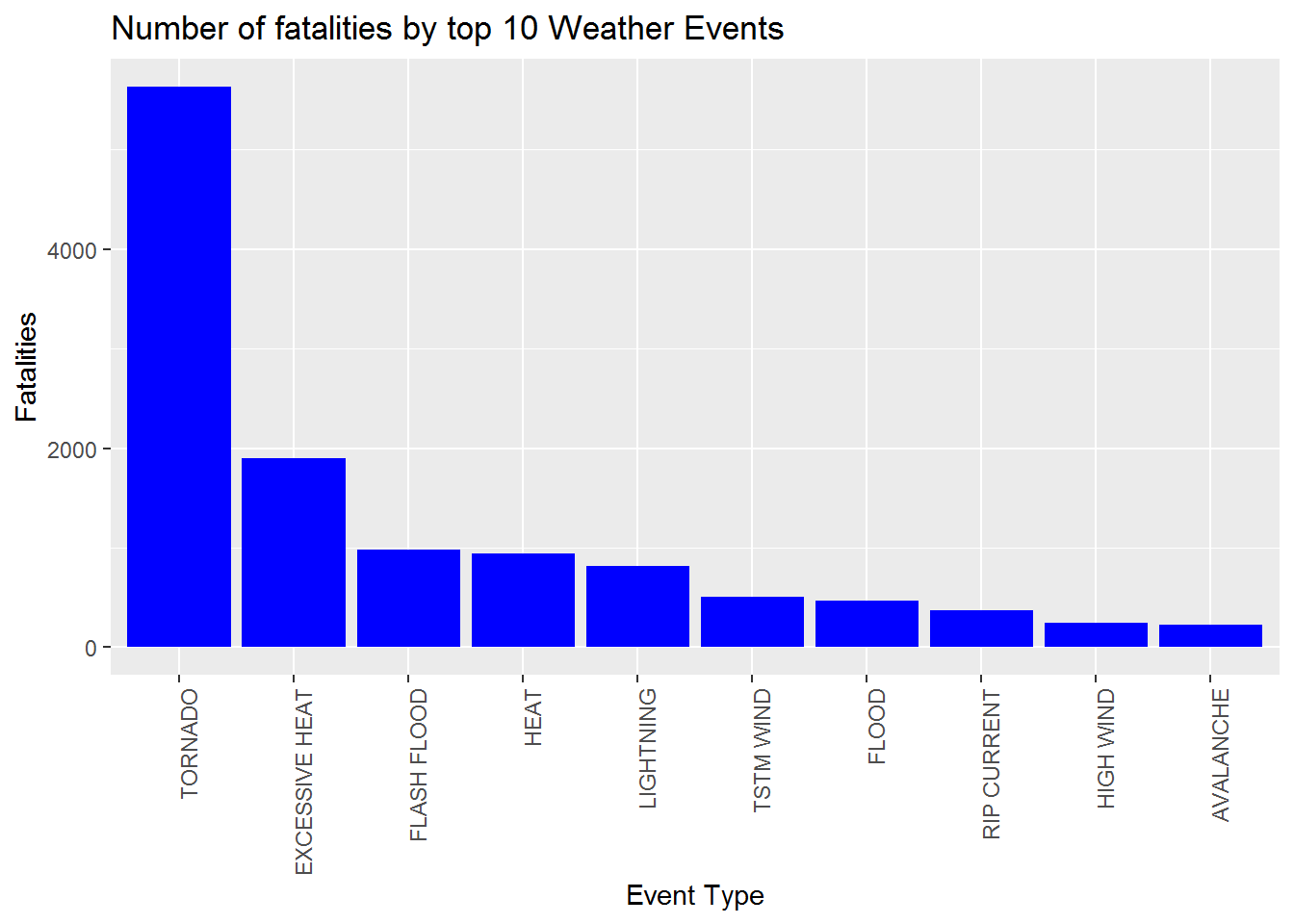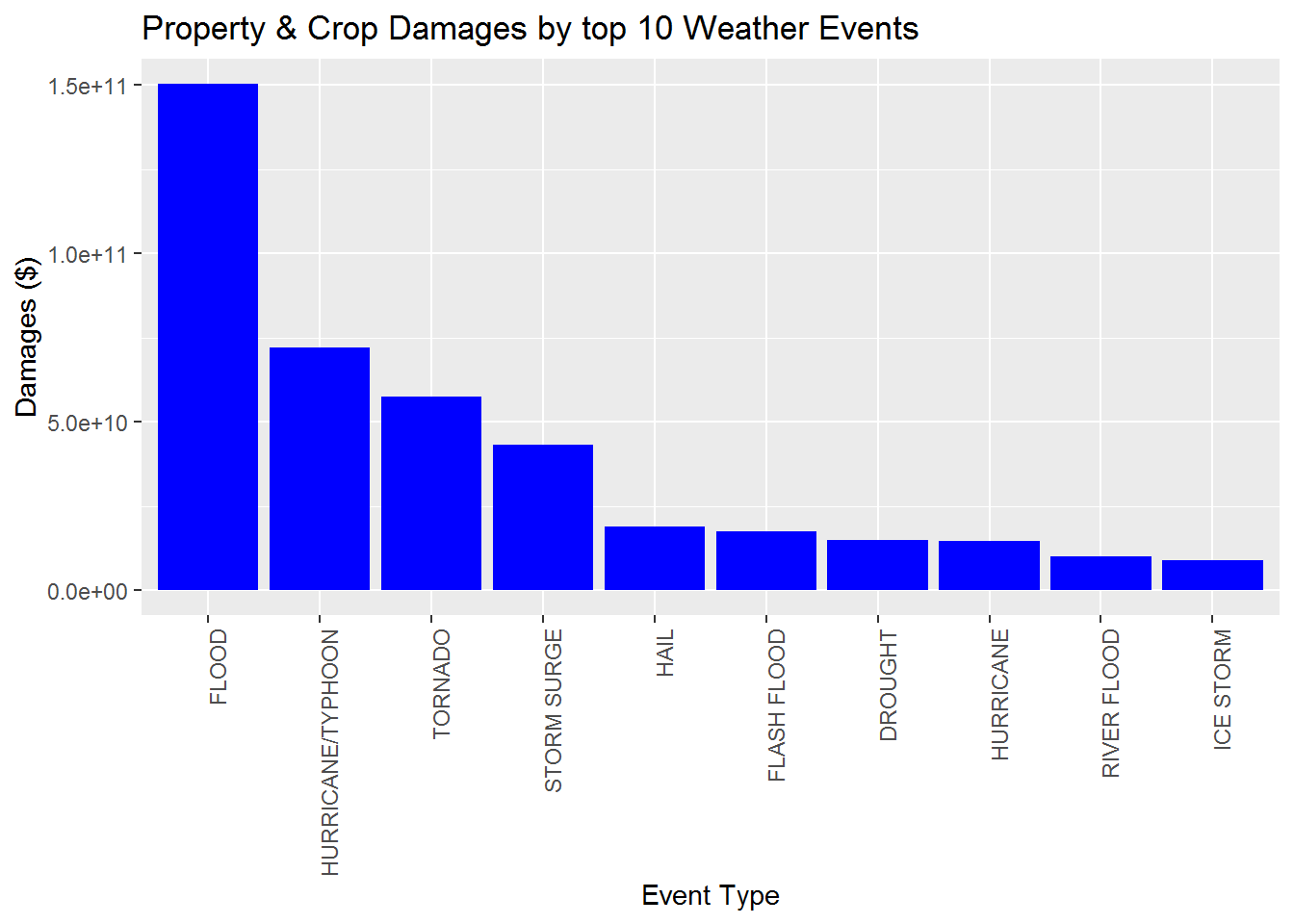# Synopsis

The goal of the assignment is to explore the NOAA Storm Database and explore the effects of storms and othere severe weather events on both population and economy. Many severe events can result in fatalities, injuries and property damande. The database starts in the year 1950 and ends in November 2011.

# Data processing

library("ggplot2")

fileUrl <- "https://d396qusza40orc.cloudfront.net/repdata%2Fdata%2FStormData.csv.bz2"
colnames(stormDF)
##   "STATE__"    "BGN_DATE"   "BGN_TIME"   "TIME_ZONE"  "COUNTY"
##   "COUNTYNAME" "STATE"      "EVTYPE"     "BGN_RANGE"  "BGN_AZI"
##  "BGN_LOCATI" "END_DATE"   "END_TIME"   "COUNTY_END" "COUNTYENDN"
##  "END_RANGE"  "END_AZI"    "END_LOCATI" "LENGTH"     "WIDTH"
##  "F"          "MAG"        "FATALITIES" "INJURIES"   "PROPDMG"
##  "PROPDMGEXP" "CROPDMG"    "CROPDMGEXP" "WFO"        "STATEOFFIC"
##  "ZONENAMES"  "LATITUDE"   "LONGITUDE"  "LATITUDE_E" "LONGITUDE_"
##  "REMARKS"    "REFNUM"

##subsetting data

tidyNOAA <- stormDF[, c("EVTYPE", "FATALITIES", "INJURIES", "PROPDMG", "PROPDMGEXP", "CROPDMG", "CROPDMGEXP")]
str(tidyNOAA)
## 'data.frame':    902297 obs. of  7 variables:
##  $EVTYPE : Factor w/ 985 levels " HIGH SURF ADVISORY",..: 834 834 834 834 834 834 834 834 834 834 ... ##$ FATALITIES: num  0 0 0 0 0 0 0 0 1 0 ...
##  $INJURIES : num 15 0 2 2 2 6 1 0 14 0 ... ##$ PROPDMG   : num  25 2.5 25 2.5 2.5 2.5 2.5 2.5 25 25 ...
##  $PROPDMGEXP: Factor w/ 19 levels "","-","?","+",..: 17 17 17 17 17 17 17 17 17 17 ... ##$ CROPDMG   : num  0 0 0 0 0 0 0 0 0 0 ...
##  $CROPDMGEXP: Factor w/ 9 levels "","?","0","2",..: 1 1 1 1 1 1 1 1 1 1 ... ##There are two variables of interest PROPDMG and CROPDMG which have great economic consequences ##Converting units to calculate Property damage tidyNOAA$PROPDMGNUM = 0
tidyNOAA[tidyNOAA$PROPDMGEXP == "H", ]$PROPDMGNUM = tidyNOAA[tidyNOAA$PROPDMGEXP == "H", ]$PROPDMG * 10^2
tidyNOAA[tidyNOAA$PROPDMGEXP == "K", ]$PROPDMGNUM = tidyNOAA[tidyNOAA$PROPDMGEXP == "K", ]$PROPDMG * 10^3
tidyNOAA[tidyNOAA$PROPDMGEXP == "M", ]$PROPDMGNUM = tidyNOAA[tidyNOAA$PROPDMGEXP == "M", ]$PROPDMG * 10^6
tidyNOAA[tidyNOAA$PROPDMGEXP == "B", ]$PROPDMGNUM = tidyNOAA[tidyNOAA$PROPDMGEXP == "B", ]$PROPDMG * 10^9

##Conerting units to calculate Crop damage

tidyNOAA$CROPDMGNUM = 0 tidyNOAA[tidyNOAA$CROPDMGEXP == "H", ]$CROPDMGNUM = tidyNOAA[tidyNOAA$CROPDMGEXP == "H", ]$CROPDMG * 10^2 tidyNOAA[tidyNOAA$CROPDMGEXP == "K", ]$CROPDMGNUM = tidyNOAA[tidyNOAA$CROPDMGEXP == "K", ]$CROPDMG * 10^3 tidyNOAA[tidyNOAA$CROPDMGEXP == "M", ]$CROPDMGNUM = tidyNOAA[tidyNOAA$CROPDMGEXP == "M", ]$CROPDMG * 10^6 tidyNOAA[tidyNOAA$CROPDMGEXP == "B", ]$CROPDMGNUM = tidyNOAA[tidyNOAA$CROPDMGEXP == "B", ]$CROPDMG * 10^9 # RESULTS 1.Events most harmful with respect to human health ##There are two variables of interest Injuries and Fatalities which are harmful to human health. we aggregate the the total injuries for each weather events library(ggplot2) totalInjuries <- aggregate(INJURIES ~ EVTYPE, data=tidyNOAA, sum) totalInjuries <- totalInjuries[order(-totalInjuries$INJURIES), ][1:10, ]
totalInjuries$EVTYPE <- factor(totalInjuries$EVTYPE, levels = totalInjuries$EVTYPE) totalFatalities <-aggregate(FATALITIES ~ EVTYPE, data = tidyNOAA, sum) totalFatalities <- totalFatalities[order(-totalFatalities$FATALITIES),  ][1:10, ]
totalFatalities$EVTYPE <- factor(totalFatalities$EVTYPE, levels = totalFatalities$EVTYPE) #Plotting number of injuries with the most harmful event type plot1 <- ggplot(totalInjuries, aes(x = EVTYPE, y = INJURIES)) + geom_bar(stat = "identity", fill = "blue") + theme(axis.text.x = element_text(angle = 90, hjust = 1)) + xlab("Event Type") + ylab("Injuries") + ggtitle("Number of injuries by top 10 Weather Events") print(plot1)#Plotting the number of facilities with the most harmful event type plot2 <- ggplot(totalFatalities, aes(x = EVTYPE, y = FATALITIES)) + geom_bar(stat = "identity", fill = "blue") + theme(axis.text.x = element_text(angle = 90, hjust = 1)) + xlab("Event Type") + ylab("Fatalities") + ggtitle("Number of fatalities by top 10 Weather Events") print(plot2)The plot reveals that Tornado are the most harmful weather events for human health. 1. Across the United States, which types of events have the greatest economic consequences? damages <- aggregate(PROPDMGNUM + CROPDMGNUM ~ EVTYPE, data=tidyNOAA, sum) names(damages) = c("EVTYPE", "TOTALDAMAGE") damages <- damages[order(-damages$TOTALDAMAGE), ][1:10, ]
damages$EVTYPE <- factor(damages$EVTYPE, levels = damages$EVTYPE) #Plotting number of damage with most harmful event type plot3 <- ggplot(damages, aes(x = EVTYPE, y = TOTALDAMAGE)) + geom_bar(stat = "identity", fill = "blue", las = 3) + theme(axis.text.x = element_text(angle = 90, hjust = 1)) + xlab("Event Type") + ylab("Damages ($)") + ggtitle("Property & Crop Damages by top 10 Weather Events")
## Warning: Ignoring unknown parameters: las
print(plot3)The plot revels that Flood have the greatest economic consequences.

# ANALYSIS

1.The plot reveals that Tornado are the most harmful weather events for human health.

2.The plot revels that Flood have the greatest economic consequences.